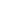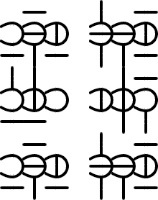This website blog chronicles my exploration into using symbology to represent alternative numerical base systems. My ultimate goal is to uncover and simplify known, and unknown, mathematical processes and connections between these systems. This is a hobby and should be taken as such. Thanks for visiting.

# 008 Three Sub-Digits of Ternary

Each trincubic numeral is really just a way to group three ternary numbers together in a compact form.
``A vertical line is used to represent 1 and a horizontal line is used to represent 2. (zero is represented as a blank area.)

A flipped C symbol is used to outline the three different positions that the ternary sub-digits can appear; below, middle and above.

This gives us 27 different combinations, 3x3x3, (3^3). It seems strange to have a number system that has an odd amount of numerals in it, but it does have some advantages worth exploring.Centipede Formation

The numerals can then be connected together to make them even more compact. Glyph connection can signify the boundaries of a complete number, or it could be used for visual separation, similar to how decimal numbers are broken up with commas every three digits.

``